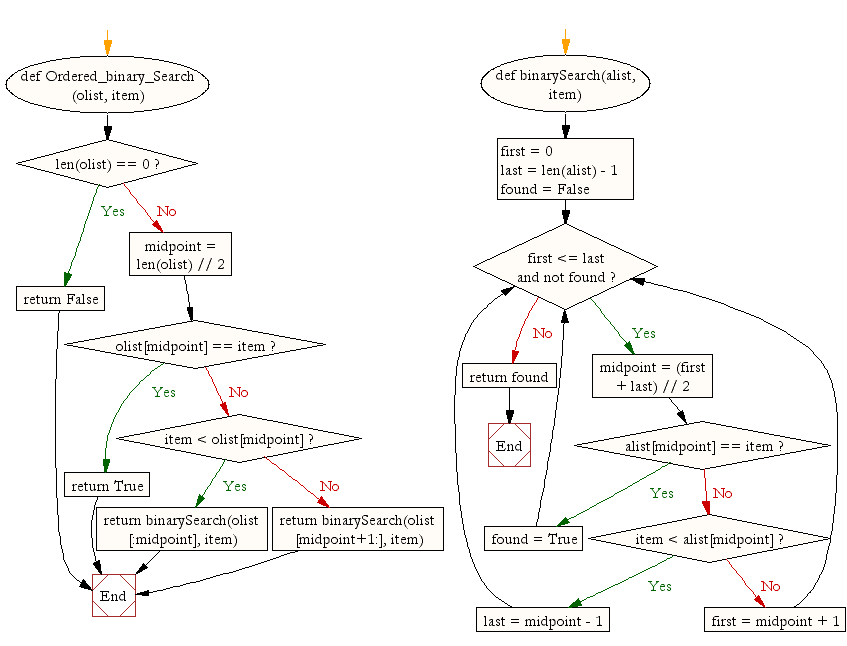﻿ Python: Search for an ordered list - w3resource# Python: Search for an ordered list

## Python Search and Sorting : Exercise-3 with Solution

Write a Python program for binary search for an ordered list.

Sample Solution:-

Python Code:

``````def Ordered_binary_Search(olist, item):

if len(olist) == 0:
return False
else:
midpoint = len(olist) // 2
if olist[midpoint] == item:
return True
else:
if item < olist[midpoint]:
return binarySearch(olist[:midpoint], item)
else:
return binarySearch(olist[midpoint+1:], item)

def binarySearch(alist, item):

first = 0
last = len(alist) - 1
found = False

midpoint = (first + last) // 2
if alist[midpoint] == item:
found = True
else:
if item < alist[midpoint]:
last = midpoint - 1
else:
first = midpoint + 1

return found

print(Ordered_binary_Search([0, 1, 3, 8, 14, 18, 19, 34, 52], 3))
print(Ordered_binary_Search([0, 1, 3, 8, 14, 18, 19, 34, 52], 17))
```
```

Sample Output:

```True
False
```

Flowchart:Python Code Editor:

What is the difficulty level of this exercise?

Test your Programming skills with w3resource's quiz.

﻿

## Python: Tips of the Day

Use Reversed() In for Loops:

```>>> tasks = ['laundry', 'picking up kids', 'gardening', 'cooking']